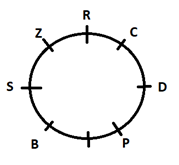# SBI Clerk/ IDBI and South Indian Bank Mains Reasoning Questions 2019 (Day-03)

Dear Aspirants, Our IBPS Guide team is providing a new series of Reasoning Questions for SBI Clerk /IDBI and South Indian Bank Mains 2019 so the aspirants can practice it on a daily basis. These questions are framed by our skilled experts after understanding your needs thoroughly. Aspirants can practice these new series questions daily to familiarize with the exact exam pattern and make your preparation effective.

Check here for SBI Clerk Mains Mock Test 2019

Check here for Repco Bank Clerk Mock Test 2019

Check here for South Indian Bank Mock Test 2019

[WpProQuiz 6712]

Directions (1-4): Refer to the data below and answer the questions that follow.

Ajay went to a bank to deposit a sum of money in his account. The banker asked for the account number from Ajay and following points are known about his account number.

Note: The account number is of 10 digits and does not start with zero. Zero is not considered as even number.

1. The last digit of the account number is divided by 3 but the digit is not 3.
2. The difference of first and last digit is greater than 6.
3. The second digit of the account number is an even prime number.
4. The 2nd, 3rd and 4th numbers are consecutive numbers starting from 2nd digit.
5. The 5th and 6th digit are even numbers greater than 4 and 6 respectively.
6. The sum of 6th and 1st digit is greater than 9.
7. The 8th digit is same as the 1st digit and 7th digit is sum of 1st and 2nd digit.
8. The 9th and 5th digits are same and are more than 7.

1) What is the 8th digit of the Ajay Account number?

a) 3

b) 4

c) 8

d) 6

e) 2

2) What is the sum of 4th 8th and 9th digit?

a) 13

b) 14

c) 18

d) 16

e) 12

3) How many even digits are there in Ajay’s Account number?

a) 6

b) 8

c) 7

d) 5

e) 9

4) What is the sum of 5th, 10th and 3rd digit?

a) 20

b) 21

c) 23

d) 19

e) 18

Directions (5-7): Refer to the data below and answer the questions that follow

Seven competitive eaters participated in eating and drinking competition. The persons who participated in both events were P, Q, R, S, T, U and V. The winner was to be selected based upon the time taken to complete the food/drinks. Each person completed their food/drinks in different time periods, i.e. no two persons completed their food/drinks at the same time. Each person obtained a particular position according to his performance. The one who got the top position performed better than others while one who got the lowest position performed worse. P ‘s position in eating competition was just above U but in drinking competition he was just below R. S ‘s position in drinking competition was just above V but in eating competition, he was just below T. No persons were assigned positions between P and S in both competitions. At least four persons got positions above P in eating competition and at least four persons are below him in drinking competition. R did not get the highest or the lowest position in any competition. U‘s performance was better than V’s in both the competitions.

5) Which of the following combination denotes the top three ranked persons in eating competition respectively?

a) Q, R and T

b) U, R and P

c) R, T and S

d) P, U and V

e) None of these

6) In drinking competition, how many persons performed better than Q?

a) Six

b) Five

c) Four

d) Can’t be determined

e) None of these

7) Who among the following got the same position both in eating and drinking competitions?

a) Only R

b) Only S

c) Both R and S

d) Can’t be determined

e) None of these

The following question consists of three statements numbered I, II and III. Decide if data given in the statements are sufficient to answer the questions below

8) Batting record of Virat, Rahane, and Rohit is compared after a test series. How many runs were scored by Rahane?

(Strike Rate = Runs Scored/Balls faced * 100)

I) The average of Rohit in 5 matches is 8 more than Rahane but 5 less than Virat.

II) Rohit scored 40 more runs than Rahane in the series.

III) Virat faced 200 balls in the series and scored at the strike rate of 162.5.

a) All the statements are needed

b) Only I and II are sufficient

c) Only II and III are sufficient

d) Only I and III are sufficient

e) Insufficient data

In the following question, a given questions is followed by information in two statements. You have to find out the data in which statement (s) is sufficient to answer the question and mark your answer accordingly.

9) Renu has 2 months semester break, at the end of each semester. In which of the 2 months she has her next semester break?

I) Each semester is of 6 months, her next semester starts in either the month of November or December.

II) Next semester start in a month of 31 days.

a) II alone is sufficient while I alone is not sufficient.

b) I alone is sufficient while II alone is not sufficient.

c) Either I or II alone is sufficient to answer the question.

d) I and II together are sufficient to answer the question.

e) Neither I nor II is sufficient to answer the question.

Each of the questions below, consist a question and three statements numbered I, II and III. You have to decide whether the data provided in the statements are sufficient to answer the question. Read the three statements and Give answer

10) If all the friends Z, B, C, D, P, Q, R and S are sitting around circular table facing outside the centre of the table, then how many persons are sitting between B and R (when counted in clockwise direction from B)?

Statements:

I) P is seated immediate right of D and third to the right of R, who is near to C.

II) S is seated third to the left of D, who is not near to Z.

III) Q is seated third to the left of Z, who is second to the right of B, who is not near to C.

a) If the data in statement I and II together are sufficient to answer the question, while the data in statement III are not required to answer the question.

b) If the data in statement I and III together are sufficient to answer the question, while the data in statement II are not required to answer the question.

c) If the data in statement II and III are sufficient to answer the question, while the data in statement I are not required to answer the question.

d) If the data in all three statements I, II and III together are necessary to answer the question.

e) If the data in all the statements, I, II and III even together are not sufficient to answer the question.

Direction (1-4):

1) The last digit of the account number is divided by 3 but the digit is not 3.

That means the digit will be 6 or 9.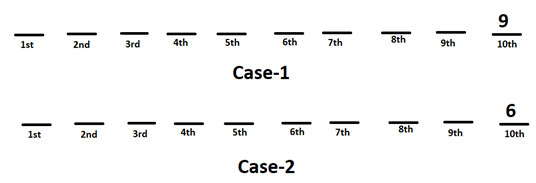2) The difference of first and last digit is greater than 6.

That means the last digit is 9 and 1st digit is 1 or 2.

3) The second digit of the account number is an even prime number.

4) The 2nd, 3rd and 4th numbers are consecutive numbers starting from 2nd digit.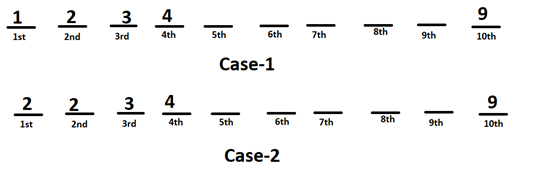5) The 5th and 6th digit are even numbers greater than 4 and 6 respectively.

It means 5th digit is 6 or 8 and 6th digit is 8.

6) The sum of 6th and 1st digit is greater than 9.

That means the 1st digit is 2.

7) The 8th digit is same as the 1st digit and 7th digit is sum of 1st and 2nd digit.

Hence 8th digit is 2 and 7th digit is 4.

8) The 9th and 5th digits are same and are more than 7.

It means 9th and 5th digits are 8 (ref point (5))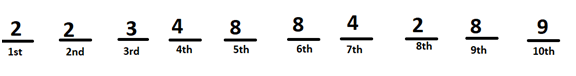Hence Ajay account number is 2234884289

Direction (5-7):

P‘s position in eating competition was just above U but in drinking competition he was just below R.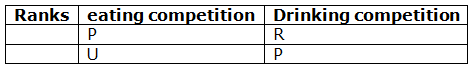S ‘s position in drinking competition was just above V but in eating competition, he was just below T. No persons were assigned positions between P and S in both competitions.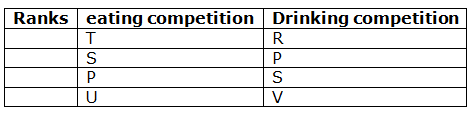At least four persons got positions above P in eating competition and at least four persons are below him in drinking competition. R did not get the highest or the lowest position in any race. U‘s performance was better than V’s in both the competitions. So, the final arrangement is—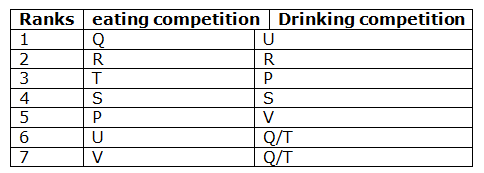Virat faced 200 balls in the series and scored at the strike rate of 162.5.

(162.5% of 200 is 325, implies Virat scored 325 runs in the series at an average of 65.)

The average of Rohit in 5 matches is 8 more than Rahane but 5 less than Virat.

(Implies, average of Rahane is 13 less than Virat i.e. 52. Means, Rahane scored 260 runs in the series.)

Hence, statement I and III are sufficient to answer the question.

From the question, Renu has two month semester break at the end of each semester and we need to find which months she has semester break.

From the statement I, Each semester is of 6 months, her next semester starts in either the month of November or December.

Above statement does not give information about the start or the end of semester. It could be either November or December.

Hence, statement I alone is not sufficient to answer the Question.

From the statement II, Next semester start in a month of 31 days.

There are more than one month which have 31 days.

Hence, statement II alone is not sufficient to answer the Question.

From the statement I and II together, Each semester is of 6 months, her next semester starts in either the month of November or December.

From the statement II, Next semester start in a month of 31 days.

December has 31 days, so semester starts in the month of December, and ends after six months. So semester ends in May.

So, semester break will be in June and July.

So, both the statements are needed for finding the answer.